# Plus One Physics Chapter Wise Questions and Answers Chapter 15 Waves

Students can Download Chapter 15 Waves Questions and Answers, Plus One Physics Chapter Wise Questions and Answers helps you to revise the complete Kerala State Syllabus and score more marks in your examinations.

## Kerala Plus One Physics Chapter Wise Questions and Answers Chapter 15 Waves

### Plus One Physics Waves One Mark Questions and Answers

Question 1.
Velocity of sound in vacuum is
(a) 330ms-1
(b) 165ms-1
(c) zero
(d) 660ms-1
(c) zero
Sound requires a material medium for propation. Hence, velocity of sound in vacuum is zero.

Question 2.
The physical quantity that remains unchanged when a sound wave goes from one medum to another is
(a) amplitude
(b) speed
(c) wavelength
(d) frequency
(d) frequency
When a sound wave goes from one medium to another the frequency of the wave remains unchanged.Question 3.
What is the range of frequency of audible sound?
20Hz to 20KHz.

Question 4.
Why does sound travel faster in iron than in air?
Because solids are more elastic compared to air.

Question 5.
What kind of waves help the bats to find their way in dark?
Ultrasonic wave

Question 6.
In which gas, hydrogen and oxygen will the sound have greater velocity?
As velocity, v α $$\sqrt{1 / ρ}$$, velocity of sound will be greater in hydrogen gas.Question 7.
Why transverse waves can not setup in gas?
The rigidity modulus of gas is zero.

Question 8.
What is the effect of pressure on the velocity of sound waves?
No effect

Question 9.
Why bells are made up of metal and not wood?
The wood causes high damping.

Question 10.
What is the velocity of sound in perfect rigid body?
The velocity is infinite cause young’s modulus of perfect rigid body is infinite.

### Plus One Physics Waves Two Mark Questions and Answers

Question 1.
If a tuning fork is held above a resonance column, then maximum sound can be heard at certain height of the air column.

1. Name the type of wave produced in the air col¬umn.
2. What do you mean by beats?

1. Longitudinal
2. Periodic variation of intensity of sound is called beats.Question 2.
Why bells of colleges and temple are of large size?
Larger, the area of source of sound more is the energy transmitted into the medium. Hence intensity of sound is more and loud sound is heard.

### Plus One Physics Waves Three Mark Questions and Answers

Question 1.
A sound wave of frequency 400 Hz is travelling in air at a speed of 320m/s.

1. The speed of sound wave in vaccum is______
• 320m/s
• more than 320m/s
• less than320m/s
• Zero
2. What is the wavelength of the above wave?
3. Calculate the diffference in phase between two points on the wave 0.2m apart in the direction of travel.

1. Zero

2. v = fλ
λ = $$\frac{v}{f}=\frac{320}{400}$$ = 0.8m

3. At 0.2 m apart, the phase differnce’is given by
∆Φ = $$\frac{0.2}{0.8} \times 2 \pi=\frac{\pi}{2} \mathrm{rad}$$.Question 2.
A string fixed one end is suddenly brought in to up and down motion.

1. What is the nature of the wane produced in the string and name the wave.
2. A brass wire 1 m long has a mass 6 × 10-3 kg. If it is kept at a tension 60N, What is the speed of the wave on the wire.

1. Transverse wave

2.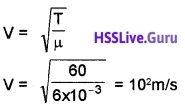### Plus One Physics Waves Four Mark Questions and Answers

Question 1.
A sonometer wire of length 30cm vibrates in the second overtone

1. Represent it pictorially
2. What is the distance between two points in the string which has a phase difference of n
3. A violin string resonates in its fundamental frequency of 196hz. Where along the string must you place your finger so that the fundamental frequency becomes 440Hz, If the length of violin string is 40cm.

1.2. 0.30 = $$\frac{3}{2}$$λ
λ = $$\frac{2 \times 0.3}{3}$$ = 0.2
We know 2π = λ
ie. π radian = $$\frac{λ}{2}$$ wave length
= $$\frac{0.2}{2}$$ = 0.1m
∴ The distance between two points is 0.1 m (for a a phase difference of π radian).

3. For fundamental mode of vibration.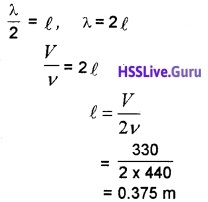The finger must be placed 37.5 from one end.Question 2.
A horizontal metal wire is fixed on a state of tension between two vertical supports when plucked it gives a fundamental frequency fO.

1. Obtain a mathematical expression for fO
2. A 5.5 m wire has a mass of 0.035 kg. If the tension of the string is 77N, the speed of wave on the string is
• 110 ms-1
• 11$$\sqrt{10}$$ms-1
• 77 ms-1
• 11 ms-1
• 102 ms-1
3. What change, if any, will be observed in the fundamental frequency if the wire is now immersed in water and plucked again?

We know velocity on a string, V = $$\sqrt{T / m}$$
But V = λf2. Mass per unit length,m = $$\frac{M}{\ell}=\frac{0.035}{5.5}$$
= 6.36 × 10-3kg/m
T = 77N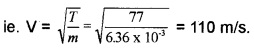3. Frequency does not change.Question 3.
When a pebble is dropped to the surface of water, certain waves are formed on the water surface.

1. What type of wave is it?
2. Is it a progressive wave? Explain?
3. The equation for such a wave is y = 4sinπ (2t – 0.01x). where ‘y’ and ‘x’ are in cm. and ‘t’ in sec. find its
• Amplitude
• Wavelength
• initial phase
• Frequency

1. Transverse wave.

2. It is a progressive wave. It moves from one point to another point.

3. y = 4sinπ(2t – 0.01x)
y = 4 sin (2πt-0.01πx)
= -4 sin (0.01 πx – 2πt)
Comparing with standard wave equation, y = A sin (kx – ωt), we get

• Amplitude A = 4 m
• Kx = 0.01 × πx
$$\frac{2 \pi}{\lambda}$$x = 0.01 πx, λ = 200m
• Initial phase = 0
• ωt = 2 πt, 2 π f t = 2 πt, f = 1 Hz.Question 4.
The speed of a wave along a stretched string depends only on the tension and the linear mass density of the string and does not dependent on the frequency of the wave.

1. Give the equation of speed of transverse wave along a stretched string.
2. Why the speed does not depend on the frequency of the wave.
3. A steel wire 0.72 long has a mass of 5 × 10-3Kg. If the wire is under a tension 60N, what is the speed of transverse wave on the wire?

1. v = $$\sqrt{\frac{T}{m}}$$ Where T is the tension and m is the mass per unit length.
2. The frequency of the wave is determined by the source that generates the wave.
3.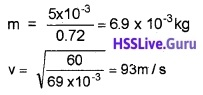Question 5.
While discussing the propagation of sound through atmospheric air, one argued that the velocity of sound is 280 ms-1 and said that he calculated it using Newton’s formula. But another learner argued that velocity of sound is 330 ms1. He justified his argument by saying that he has applied Laplace corrected formula.

1. Write the formula used by the second learner.
2. Using the above relation, show that velocity depends on temperature and humidity while is independent of pressure.
3. “Sound can be heard over longer distance on rainy days.” Justify.

1. a = $$\sqrt{\frac{2 \lambda P}{\rho}}$$

2.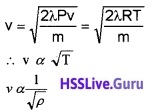If temperature remain same
PV = constant
∴ v is independent of p.

3. During rainy day as ρ decreases. Hence v increase and sound propagate longer distance.Question 6.
When a stone is dropped in to the river, certain waves are formed on its surface.

1. What type of wave it is?
2. Is it a progressive wave? Explain.
3. If yes, derive a mathematical expression for the above wave.

1. Transverse wave.

2. Yes, because each particles of the medium vi¬brates simple harmonically.

3. Consider a harmonic wave travelling along the +ve x-direction with a speed V. Let ‘0’ be the particle in the medium. Its displacement at any instant of time may be written as y = A sin ωtConsider another particle ‘p’ at a distance x from ‘0’ to its right. The displacement of p at any instant.
y = A sin (ωt – α) ______(1)
α is the phase difference between 0 and P. Here
α = $$\frac{2 \pi}{\lambda}$$x equation (1) becomes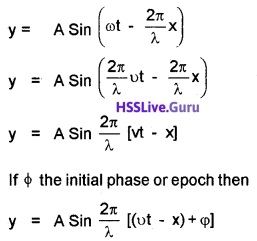### Plus One Physics Waves Five Mark Questions and Answers

Question 1.
A boy standing near a railway track found that the pitch of the siren of a train increases as it approaches him

1. State the phenomenon behind it?
2. List any two applications of the same Phenomenon
3. Obtain an expression for the apparent frequency of the siren as heard by the boy.

1. Doppler effect.

2. Doppler effect can be used to find the speed of moving object. Dopplar effect in light is used to find speed of galaxies.

3. The apparent change in the frequency of sound wave due to the relative motion of source or listener or both is called Doppler effect. It was proposed by John Christian Doppler and it was experimentally tested by Buys Ballot.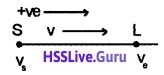Considers source is producing sound of frequency v. Let V be the velocity of sound in the medium and λ the wavelength of sound when the source and the listener are at rest.

The frequency of sound heard by the listener is ν = $$\frac{v}{\lambda}$$. Let the source and listener be moving with velocities vs and vl in the direction of propogation of sound from source to listener. (The direction S to L is taken as positive).The relative velocity of sound wave with respect to the source = V – Vs
Apparent wavelength of sound,
λ1 = $$\frac{V-V_{s}}{v}$$ _____(1)
Since the listener is moving with velocity v^, the relative velocity of sound with respect to the listener,
V1 = V – Vl ______(2)
Apparent frequency of sound as heard by the listener is given by
ν = $$\frac{v^{1}}{\lambda^{1}}$$ ______(3)
Sub (1) and (2) in eq.(3) we getQuestion 2.

1. Waves are means of transferring energy from one point to another. Distinguish between longitudinal and transverse waves.
2. What is a plane progressive wave? Arrive at an expression for the displacement of a particle on the path of the wave, advancing in the positive x-direction.
3. The velocity of sound is greater in solids than in gases. Explain.

1.

 Longitudinal wave Transverse wave 1. Can’t be polarized 2. Direction of propagation is parallel to the direction of vibration of particles. 1. Can be polarized 2. Direction of propagation is perpendicular to the direction of vibration of particles.

2. Out of syllabus

3. Solids are highly elastic as compared to liquids and gases.Question 3.

1. Sound produced by an open pipe contains:
• Fundamental component only
• Odd harmonics only
• All the harmonics
• Even harmonics only
2. A pipe 30 cm long is open at both ends. Which harmonic mode of the pipe is resonantly exerted by a 1.1 kHz source?
3. Will resonance with the same source be observed if one end of the pipe is closed? (Take the speed
of sound in air to be 330 ms-1)

1. All the harmonics.

2. We know frequency of oscillation in the Pipe, f = $$\frac{n V}{2 L}$$
Substituting the values v, L and f we getn = 2 means that the oscillation is second harmonics.

3. The condition for second harmonics in closed pipe is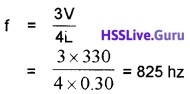The frequency required for resonance in a closed pipe is 825 Hz. Hence we do not get resonance at 1.1 Khz.Question 4.
A boy plucks at the centre of a stretched string of length 1 m and abserves a wave pattern.

1. Which type of wave is produced on the string?
2. What are the conditions for the formation of the above mentioned wave?
3. The distance between consecutive nodes is
1. λ
2. λ/2
3. λ/4
4. A steel rod100 cm long is clamped at its middle. The fundamental frequency of longitudiral vibrations of the rod is given to be 2.5kHz. What is the speed of sound in steel?

1. Standing wave (or) stationary wave

2. Same frequency, same amplitude, travelling in opposite direction.

3. λ/2

4. Length of the rod l = $$\frac{\lambda_{1}}{2}$$
λ1 = 2l =2m
v = ν1λ1
v = 2500 × 2 = 5000 m/s.

### Plus One Physics Waves NCERT Questions and Answers

Question 1.
A string of mass 2.50kg is under a tension of200N. The length of the stretched string is 20.0m. If the transverse jerk is struck at one end of the string, how long does the disturbance take to reach the other end?
Tension T = 200N
Length I = 20.0m; Mass M = 2.50kgQuestion 2.
A stone droped from the top of a tower of height 300m high splashes into the water of a pond near the base of the tower. When is the splash heard at the top. Given that the speed of sound in air is 340ms-1? (g = 9.8ms-2)
Time after which the splash is heard at the top is equal to the sum of the time t1 taken by the stone to fall down and the time t2 taken by the sound to travel from bottom to top.
Using S = ut + $$\frac{1}{2}$$ at2, we 9et S = $$\frac{1}{2}$$gt12
(∵ u = 0 and a =g)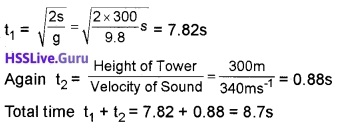Question 3.
A hospital uses an ultrasonic scanner to locate tumours in a tissue. What is the wavelength of sound in the tissue in which the speed of sound is 1.7kms-1? The operating frequency of the scanner is 4.2MHz.
λ = ? u = 1.7kms-1 = 1700ms-1
v = 4.2 × 106 Hz, u = vλ = or λ = $$\frac{u}{v}$$
or λ = $$\frac{1700}{4.2 \times 10^{6}}$$ m = 4.05 × 10-4 m = 0.405mm.Question 4.
A wire stretched between two rigid supports vibrates in its fundamental mode with a frequency of 45Hz. The mass of the wire is 3.5 × 10-2kg and its linear mass density is 4.0 × 10-2kgm-1. What is

1. the speed of a transverse wave on the string and
2. the tension in the string?

mass of wire M = 3.5 × 10-2kg
Linear density µ = mass / length
= M/l = 4.0 × 10-2kg
∴ Length of wire l = $$\frac{M}{\mu}=\frac{3.5 \times 10^{-2}}{4 \times 10^{-2}}$$ m = 0.875m
In the fundamental mode,
λ =2l = 2 × 0.875m = 1.75m

1. Speed of transverse vyaves u = v λ
= 45 × 1.75ms-1 = 78.75ms-1

2. u= $$\sqrt{\frac{T}{\mu}}$$ or T = µu2 = 4 × 10-2(78.75)2N
= 278.06N.Question 5.
A steel rod 100cm long is clamped at its middle. The fundemental frequency of longitudinal vibrations of the rod are given to be 2.53kHz. What is the speed ‘ of sound in steel?
l = 1m, v = 2.53 × 103HZ, $$\frac{\lambda}{2}$$ = I or λ = 2m